# 面试必考题——递归解题套路## 导航

• 前言
• 什么是递归
• 递归算法通用解决思路
• 实战演练（从初级到高阶）
• 热身赛
• 入门题
• 初级题
• 中级题
• 进阶题
• 结语

递归是算法中一种非常重要的思想,应用也很广。

• 有很多数学函数是递归定义的,如大家熟悉的阶乘函数,2阶Fibonacci数列和Ackerman函数。
• 有的数据结构,如二叉树,广义表等,由于结构本身固有的递归特性,则它们的操作可以递归描述。
• 还有一类问题,虽然问题本身没有明显的递归结构，但用递归求解比迭代更为简单,如八皇后问题,Hanoi塔问题。

### 前言

最近看了不少递归的文章,不过我发现大部分网上讲递归的文章都不太全面,主要的问题在于解题后大部分都没有给出相应的时间/空间复杂度,而时间/空间复杂度是算法的重要考量!递归算法的时间复杂度普遍比较难(需要用到归纳法等)。换句话说,如果能解决递归的算法复杂度,其他算法题题的时间复杂度也基本不在话下。另外,递归算法的时间复杂度不少是不能接受的,如果发现算出的时间复杂度过大,则需要转换思路,看下是否有更好的解法,这才是根本目的,不要为了递归而递归

本文试图从以下几个方面来讲解递归:

1. 什么是递归？
2. 递归算法通用解决思路
3. 实战演练（从初级到高阶）

力争让大家对递归的认知能上一个新台阶,特别会对递归的精华:时间复杂度作详细剖析，会给大家总结一套很通用的求解递归时间复杂度的套路，相信你看完肯定会有收获。

### 什么是递归

一个直接调用自己或者通过一系列的调用语句间接地调用自己的函数,称作递归函数。递归是程序设计一个强有力的工具。

以阶乘函数为例,如下,在 factorial 函数中存在着 factorial(n - 1) 的调用，所以此函数是递归函数

public int factorial(int n) {
if (n < =1) {
return 1;
}
return n * factorial(n - 1)
}


进一步剖析「递归」，先有「」再有「」，「」的意思是将问题拆解成子问题来解决， 子问题再拆解成子子问题，...，直到被拆解的子问题无需再拆分成更细的子问题（即可以求解），「」是说最小的子问题解决了，那么它的上一层子问题也就解决了，上一层的子问题解决了，上上层子问题自然也就解决了,....,直到最开始的问题解决,文字说可能有点抽象，那我们就以阶层f(6)为例来看下它的「」和「」。是不是有点微积分的思想,哈哈...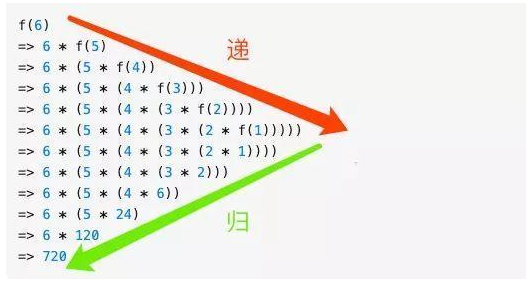求解问题f(6),由于f(6)=n*f(5),所以f(6)需要拆解成f(5)子问题进行求解,同理f(5)= n * f(4)
,也需要进一步拆分,... ,直到 f(1), 这是「」，f(1) 解决了，由于 f(2) = 2 f(1) = 2 也解决了,.... f(n)到最后也解决了，这是「」，所以递归的本质是能把问题拆分成具有相同解决思路的子问题...直到最后被拆解的子问题再也不能拆分，解决了最小粒度可求解的子问题后，在「」的过程中自然顺其自然地解决了最开始的问题。

### 递归算法通用解决思路

我们在上一节仔细剖析了什么是递归,可以发现递归有以下两个特点

• 一个问题可以分解成具有相同解决思路的子问题,子子问题,换句话说这些问题都能调用同一个函数
• 经过层层分解的子问题最后一定是有一个不能再分解的固定值的(即终止条件),如果没有的话,就无穷无尽地分解子问题了,问题显然是无解的

所以解递归题的关键在于我们首先需要根据以上递归的两个特点判断题目是否可以用递归来解。

经过判断可以用递归后，接下来我们就来看看用递归解题的基本套路(四步曲):

1. 先定义一个函数，明确这个函数的功能，由于递归的特点是问题和子问题都会调用函数自身，所以这个函数的功能一旦确定了， 之后只要找寻问题与子问题的递归关系即可
2. 接下来寻找问题与子问题间的关系（即递推公式），这样由于问题与子问题具有相同解决思路，只要子问题调用步骤 1 定义好的函数，问题即可解决。所谓的关系最好能用一个公式表示出来，比如 f(n) = n * f(n-1) 这样，如果暂时无法得出明确的公式，用伪代码表示也是可以的, 发现递推关系后，要寻找最终不可再分解的子问题的解，即（临界条件），确保子问题不会无限分解下去。由于第一步我们已经定义了这个函数的功能，所以当问题拆分成子问题时，子问题可以调用步骤 1 定义的函数，符合递归的条件（函数里调用自身）
3. 将第二步的递推公式用代码表示出来补充到步骤 1 定义的函数中
4. 最后也是很关键的一步，根据问题与子问题的关系，推导出时间复杂度,如果发现递归时间复杂度不可接受，则需转换思路对其进行改造，看下是否有更靠谱的解法

听起来是不是很简单，接下来我们就由浅入深地来看几道递归题，看下怎么用上面的几个步骤来套。

### 实战演练(从初级到高阶)

#### 热身赛

输入一个正整数n，输出n!的值。其中n!=123…n,即求阶乘



套用上一节我们说的递归四步解题套路来看看怎么解。

1. 定义这个函数，明确这个函数的功能，我们知道这个函数的功能是求n的阶乘, 之后求n-1,n-2的阶乘就可以调用此函数了
/**
* 求 n 的阶乘
*/
public int factorial(int n) {
}


1. 寻找问题与子问题的关系 阶乘的关系比较简单， 我们以 f(n) 来表示n的阶乘,显然f(n)= n * f(n - 1), 同时临界条件是 f(1) = 1,即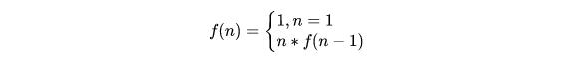1. 将第二步的递推公式用代码表示出来补充到步骤 1 定义的函数中
**
* 求 n 的阶乘
*/
public int factorial(int n) {
// 第二步的临界条件
if (n < =1) {
return 1;
}

// 第二步的递推公式
return n * factorial(n-1)
}

1. 求时间复杂度 由于 f(n) = n * f(n-1) = n * (n-1) * .... * f(1),总共作了n次乘法,所以时间复杂度为 n。

看起来是不是有这么点眉目，当然这道题确实太过简单,很容易套路，那我们再来看进阶一点的题

#### 入门题

一只青蛙可以一次跳 1 级台阶或一次跳 2 级台阶,例如:


1. 定义一个函数，这个函数代表了跳上 n 级台阶的跳法
/**
* 跳 n 极台阶的跳法
*/
public int f(int n) {
}


寻找问题与子问题之前的关系 这两者之前的关系初看确实看不出什么头绪,但仔细看题目,一只青蛙只能跳一步或两步台阶,自上而下地思考,也就是说如果要跳到n级台阶只能从n-1 或n-2 级跳， 所以问题就转化为跳上n-1和n-2级台阶的跳法了,如果f(n)代表跳到n级台阶的跳法,那么从以上分析可得f(n)=f(n-1)+f(n-2),显然这就是我们要找的问题与子问题的关系,而显然当n=1,n=2,即跳一二级台阶是问题的最终解,于是递推公式系为1. 将第二步的递推公式用代码表示出来补充到步骤 1 定义的函数中 补充后的函数如下
/**
* 跳 n 极台阶的跳法
*/
public int f(int n) {
if (n == 1) return 1;
if (n == 2) return 2;
return f(n-1) + f(n-2)
}

1. 计算时间复杂度 由以上的分析可知 f(n) 满足以下公式斐波那契的时间复杂度计算涉及到高等代数的知识,这里不做详细推导,我们直接结出结论由此可知时间复杂度是指数级别,显然不可接受,那回过头来看为啥时间复杂度这么高呢,假设我们要计算f(6),根据以上推导的递归公式,展示如下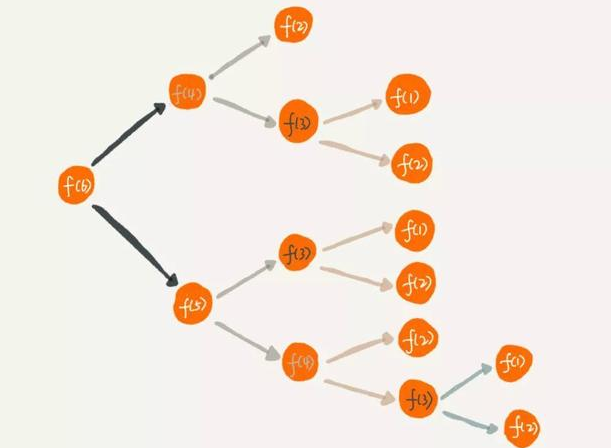可以看到有大量的重复计算, f(3)计算了3次,随着n的增大,f(n)的时间复杂度自然呈指数上升了

1. 优化

既然有这么多的重复计算，我们可以想到把这些中间计算过的结果保存起来，如果之后的计算中碰到同样需要计算的中间态，直接在这个保存的结果里查询即可，这就是典型的 以空间换时间,改造后的代码如下

public int f(int n) {
if (n == 1) return 1;
if (n == 2) return 2;
// map 即保存中间态的键值对， key 为 n，value 即 f(n)
if (map.get(n)) {
return map.get(n)
}
return f(n-1) + f(n-2)
}


那么改造后的时间复杂度是多少呢,由于对每一个计算过的f(n)我们都保存了中间态,不存在重复计算的问题,所以时间复杂度是O(n),但由于我们用了一个键值对来保存中间的计算结果,所以空间复杂度是 O(n)。问题到这里其实已经算解决了,但身为有追求的程序员,我们还是要问一句,空间复杂度能否继续优化?

使用循环迭代来改造算法 我们在分析问题与子问题关系（f(n) = f(n-1) + f(n-2)）的时候用的是自顶向下的分析方式,但其实我们在解 f(n) 的时候可以用自下而上的方式来解决，通过观察我们可以发现以下规律

f(1) = 1
f(2) = 2
f(3) = f(1) + f(2) = 3
f(4) = f(3) + f(2) = 5
....
f(n) = f(n-1) + f(n-2)


最底层f(1),f(2)的值是确定的,之后的f(3),f(4),...等问题都可以根据前两项求解出来,一直到f(n)。所以我们的代码可以改造成以下方式

public int f(int n) {
if (n == 1) return 1;
if (n == 2) return 2;

int result = 0;
int pre = 1;
int next = 2;

for (int i = 3; i < n + 1; i ++) {
result = pre + next;
pre = next;
next = result;
}
return result;
}


改造后的时间复杂度是 O(n), 而由于我们在计算过程中只定义了两个变量（pre，next），所以空间复杂度是O(1)

简单总结一下:分析问题我们需要采用自上而下的思维,而解决问题有时候采用自下而上的方式能让算法性能得到极大提升,思路比结论重要

#### 初级题

接下来我们来看下一道经典的题目:反转二叉树 将左边的二叉树反转成右边的二叉树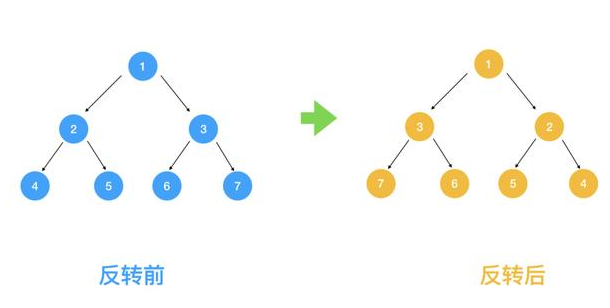接下来让我们看看用我们之前总结的递归解法四步曲如何解题

public static class TreeNode {
int val;
TreeNode left;
TreeNode right;
TreeNode(int x) { val = x; }
}

public TreeNode invertTree(TreeNode root) {
}


1. 定义一个函数，这个函数代表了翻转以 root 为根节点的二叉树
public static class TreeNode {
int val;
TreeNode left;
TreeNode right;
TreeNode(int x) { val = x; }
}

public TreeNode invertTree(TreeNode root) {
}

1. 查找问题与子问题的关系,得出递推公式 我们之前说了,解题要采用自上而下的思考方式,那我们取前面的1,2,3结点来看,对于根节点1来说,假设2,3结点下的节点都已经翻转,那么只要翻转2,3节点即满足需求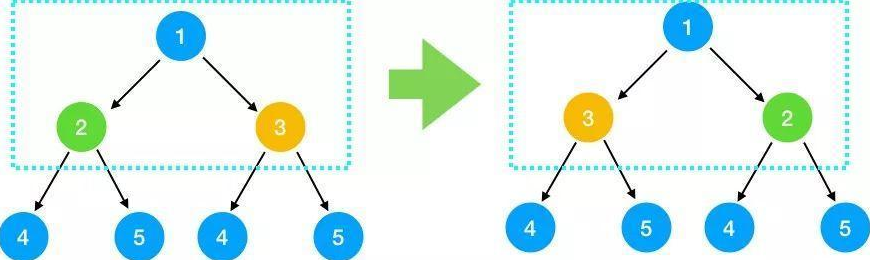对于2,3结点来说,也是翻转其左右节点即可,依此类推,对每一个根节点,依次翻转其左右节点,所以我们可知问题与子问题的关系是 翻转(根节点) = 翻转(根节点的左节点)+翻转(根节点的右节点) 即

invert(root) = invert(root->left) + invert(root->right)


而显然递归的终止条件是当结点为叶子结点时终止（因为叶子节点没有左右结点

1. 将第二步的递推公式用代码表示出来补充到步骤 1 定义的函数中
public TreeNode invertTree(TreeNode root) {
// 叶子结果不能翻转
if (root == null) {
return null;
}
// 翻转左节点下的左右节点
TreeNode left = invertTree(root.left);
// 翻转右节点下的左右节点
TreeNode right = invertTree(root.right);

// 左右节点下的二叉树翻转好后，翻转根节点的左右节点
root.right = left;
root.left = right;
return root;
}


4.时间复杂度分析 由于我们会对每一个节点都去做翻转,所以时间复杂度是O(n),那么空间复杂度呢,这道题的空间复杂度非常有意思,我们一起来看下,由于每次调用invertTree函数都相当于一次压栈操作,那最多压了几次栈呢,仔细看上面函数的下一段代码

TreeNode left = invertTree(root.left);


从根节点出发不断对左结果调用翻转函数,直到叶子节点,每调用一次都会压栈,左节点调用完后,出栈,再对右节点压栈....,下图可知栈的大小为3，即树的高度，如果是完全二叉树,则树的高度为logn,即空间复杂度为O(logn)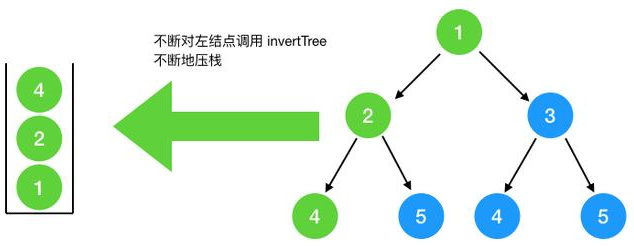最坏情况,如果此二叉树是如图所示(只有左节点,没有右节点),则树的高度即结点的个数n,此时空间复杂度为 O(n),总的来看,空间复杂度为O(n)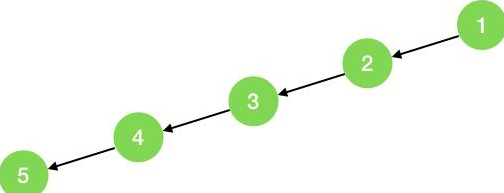#### 中级题

接下来我们看一下大学时学过的汉诺塔问题:如下图所示,从左到右有A、B、C三根柱子,其中A柱子上面有从小叠到大的n个圆盘,现要求将A柱子上的圆盘移到C柱子上去,期间只有一个原则：一次只能移到一个盘子且大盘子不能在小盘子上面，求移动的步骤和移动的次数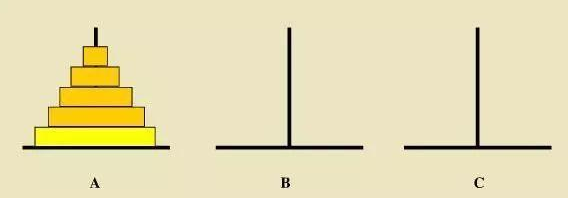接下来套用我们的递归四步法看下这题怎么解

1. 定义问题的递归函,明确函数的功能,我们定义这个函数的功能为:把A上面的n个圆盘经由B移到C
// 将 n 个圆盘从 a 经由 b 移动到 c 上
public void hanoid(int n, char a, char b, char c) {
}

1. 查找问题与子问题的关系 首先我们看如果A柱子上只有两块圆盘该怎么移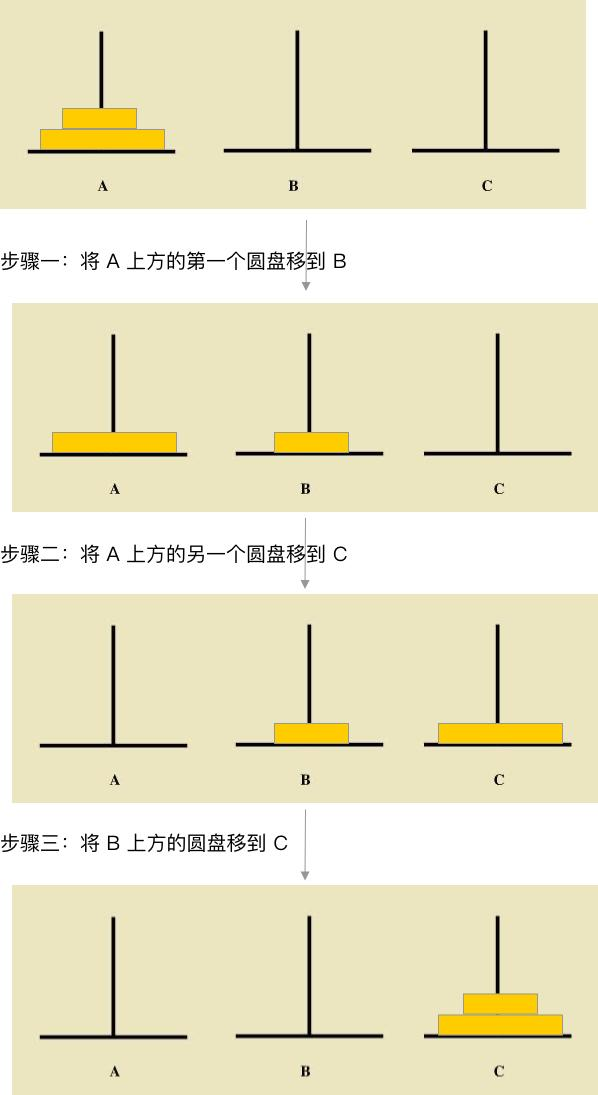前面我们多次提到，分析问题与子问题的关系要采用自上而下的分析方式,要将n个圆盘经由B移到C柱上去,可以按以下三步来分析

a. 将上面的n-1个圆盘看成是一个圆盘,这样分析思路就与上面提到的只有两块圆盘的思路一致了

b. 将上面的n-1个圆盘经由C移到B,此时将 A 底下的那块最大的圆盘移到C

c. 再将B上的n-1个圆盘经由A移到C上

有人问第一步的n-1怎么从C移到B,重复上面的过程,只要把上面的n-2个盘子经由A移到B,再把A最下面的盘子移到C,最后再把上面的n-2的盘子经由A移到B下..., 怎么样,是不是找到规律了,不过在找问题的过程中切忌把子问题层层展开,到汉诺塔这个问题上切忌再分析n-3,n-4 怎么移,这样会把你绕晕,只要找到一层问题与子问题的关系得出可以用递归表示即可。

由以上分析可得

move(n from A to C) = move(n-1 from A to B) + move(A to C) + move(n-1 from B to C)


一定要先得出递归公式，哪怕是伪代码也好!这样第三步推导函数编写就容易很多,终止条件我们很容易看出,当 A上面的圆盘没有了就不移了

• 根据以上的递归伪代码补充函数的功能
// 将 n 个圆盘从 a 经由 b 移动到 c 上
public void hanoid(int n, char a, char b, char c) {
if (n <= 0) {
return;
}
// 将上面的 n-1 个圆盘经由 C 移到 B
hanoid(n-1, a, c, b);
// 此时将 A 底下的那块最大的圆盘移到 C
move(a, c);
// 再将 B 上的 n-1 个圆盘经由A移到 C上
hanoid(n-1, b, a, c);
}

public void move(char a, char b) {
printf("%c->%c\n", a, b);
}


从函数的功能上看其实比较容易理解，整个函数定义的功能就是把 A 上的 n 个圆盘 经由 B 移到 C，由于定义好了这个函数的功能，那么接下来的把 n-1 个圆盘 经由 C 移到 B 就可以很自然的调用这个函数,所以明确函数的功能非常重要,按着函数的功能来解释，递归问题其实很好解析，切忌在每一个子问题上层层展开死抠,这样这就陷入了递归的陷阱，计算机都会栈溢出，何况人脑

时间复杂度分析 从第三步补充好的函数中我们可以推断出

f(n) = f(n-1) + 1 + f(n-1) = 2f(n-1) + 1 = 2(2f(n-2) + 1) + 1 = 2 * 2 * f(n-2) + 2 + 1 = 22 * f(n-3) + 2 + 1 = 22 * f(n-3) + 2 + 1 = 22 * (2f(n-4) + 1) = 23 * f(n-4) + 22 + 1 = .... // 不断地展开 = 2n-1 + 2n-2 + ....+ 1


显然时间复杂度为O(2n)，很明显指数级别的时间复杂度是不能接受的，汉诺塔非递归的解法比较复杂，大家可以去网上搜一下

#### 进阶题

实际面试中,很多递归题都不会用上面这些相对比较容易理解的题,更加地是对递归问题进行相应地变形,来看下面这道题

细胞分裂 有一个细胞 每一个小时分裂一次，一次分裂一个子细胞，第三个小时后会死亡。那么n个小时候有多少细胞？


照例,我们用前面的递归四步曲来解

1. 定义问题的递归函数,明确函数的功能 我们定义以下函数为n个小时后的细胞数
public int allCells(int n) {
}

1. 接下来寻找问题与子问题间的关系（即递推公式）首先我们看一下一个细胞出生到死亡后经历的所有细胞分裂过程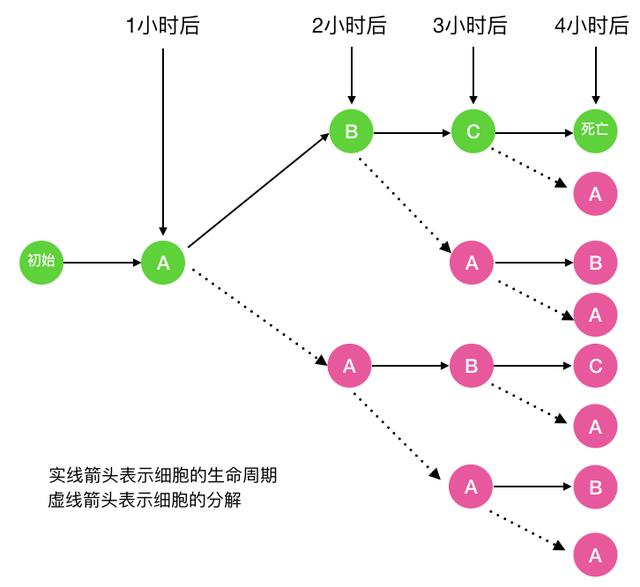图中的A代表细胞的初始态, B代表幼年态(细胞分裂一次),C代表成熟态(细胞分裂两次),C再经历一小时后细胞死亡 以f(n)代表第n小时的细胞分解数fa(n) 代表第n小时处于初始态的细胞数,fb(n)代表第n小时处于幼年态的细胞数fc(n) 代表第 n 小时处于成熟态的细胞数 则显然 f(n) = fa(n) + fb(n) + fc(n) 那么 fa(n) 等于多少呢，以n = 4 （即一个细胞经历完整的生命周期）为例

仔细看上面的图,可以看出 fa(n)=fa(n-1) + fb(n-1) + fc(n-1), 当 n = 1 时，显然 fa(1) = 1

fb(n) 呢,看下图可知 fb(n) = fa(n-1)。当 n = 1 时 fb(n) = 0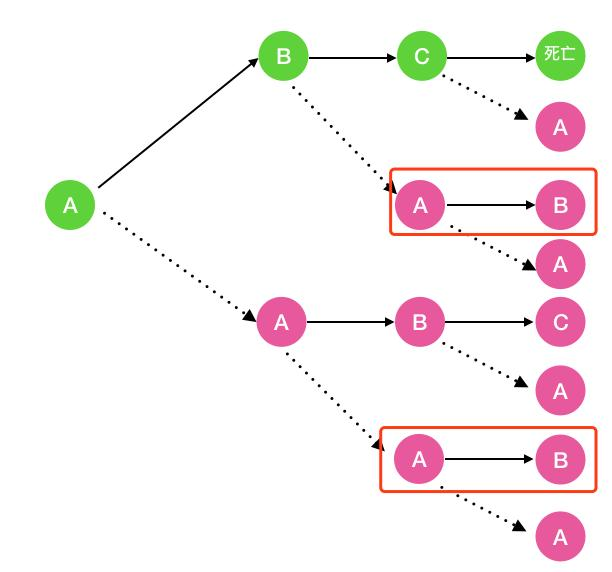fc(n)呢,看下图可知 fc(n) = fb(n-1)。当n = 1,2 时fc(n) = 0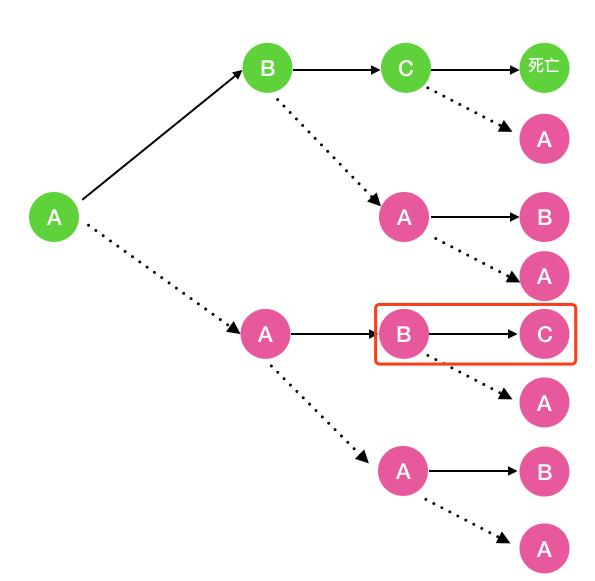综上,我们得出的递归公式如下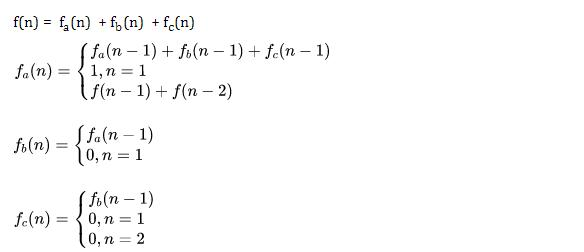根据以上递归公式我们补充一下函数的功能

public int allCells(int n) {
return aCell(n) + bCell(n) + cCell(n);
}

/**
* 第 n 小时 a 状态的细胞数
*/
public int aCell(int n) {
if(n==1){
return 1;
}else{
return aCell(n-1)+bCell(n-1)+cCell(n-1);
}
}

/**
* 第 n 小时 b 状态的细胞数
*/
public int bCell(int n) {
if(n==1){
return 0;
}else{
return aCell(n-1);
}
}

/**
* 第 n 小时 c 状态的细胞数
*/
public int cCell(int n) {
if(n==1 || n==2){
return 0;
}else{
return bCell(n-1);
}
}
`

只要思路对了,

,另一方面也告诉我们，可能一时的递归关系我们看不出来,此时可以借助于画图来观察规律

1. 求时间复杂度 由第二步的递推公式我们知道 f(n) = 2aCell(n-1) + 2aCell(n-2) + aCell(n-3)

之前青蛙跳台阶时间复杂度是指数级别的，而这个方程式显然比之前的递推公式(f(n) = f(n-1) + f(n-2)) 更复杂的，所以显然也是指数级别的

### 结语

大部分递归题其实还是有迹可寻的,按照之前总结的解递归的四个步骤可以比较顺利的解开递归题,一些比较复杂的递归题我们需要勤动手,画画图,观察规律,这样能帮助我们快速发现规律,得出递归公式,一旦知道了递归公式,将其转成递归代码就容易多了,很多大厂的递归考题并不能简单地看出递归规律,往往会在递归的基础上多加一些变形,不过万遍不离其宗，我们多采用自顶向下的分析思维,多练习，相信递归不是什么难事。

• [x] 好文同步更新到我的站点智客坊

### 参考posted @ 2019-12-16 12:01  戎"码"一生  阅读(757)  评论(1编辑  收藏